Courses

# Test: RCC Design- 1

## 10 Questions MCQ Test RRB JE for Civil Engineering | Test: RCC Design- 1

Description
This mock test of Test: RCC Design- 1 for Civil Engineering (CE) helps you for every Civil Engineering (CE) entrance exam. This contains 10 Multiple Choice Questions for Civil Engineering (CE) Test: RCC Design- 1 (mcq) to study with solutions a complete question bank. The solved questions answers in this Test: RCC Design- 1 quiz give you a good mix of easy questions and tough questions. Civil Engineering (CE) students definitely take this Test: RCC Design- 1 exercise for a better result in the exam. You can find other Test: RCC Design- 1 extra questions, long questions & short questions for Civil Engineering (CE) on EduRev as well by searching above.
QUESTION: 1

### Consider the Exposure condition as "Concrete surfaces protected against weather or aggressive conditions, except those situated in coastal areas". As per IS 456:2000, The above exposure condition will correspond to:

Solution:

As per clause 8.2.2.1, Table No 3 of IS 456:2000,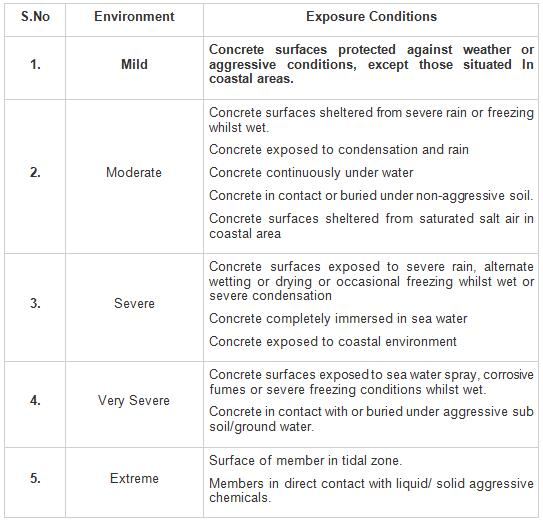QUESTION: 2

### As per IS 456:2000, the diagonal tension failure in RCC beams depends upon:

Solution:

The allowable shear stress (design shear strength) depends on both percentage of longitudinal steel provided and grade of concrete used in the beam.

QUESTION: 3

### Beam sections designed in accordance with LSM as compared to sections designed in accordance with WSM will have

Solution:

Balanced Sections designed on the basis of LSM give smaller depth or width (less concrete area) and more reinforcement than those designed with WSM.

QUESTION: 4

Minimum shear reinforcement in beams is provided in the form of stirrups

Solution:

The minimum shear reinforcement is provided for the following:

(i) Any sudden failure of beams is prevented if concrete cover bursts and the bond to the tension steel is lost.

(ii) Brittle shear failure is arrested which would have occurred due to principal tension without shear reinforcement.

(iii) Tension failure is prevented which would have occurred due to shrinkage, thermal stresses and internal cracking in beams.

(iv) To hold the reinforcement in place when concrete is poured.

(v) Section becomes effective with the tie effect of the compression steel.

QUESTION: 5

Consider the following statements and Identify the correct option with regard to provisions of IS 456: 2000:

P. The minimum stripping time for props to slab spanning over 4.5 m is 7 days

Q. The minimum stripping time for props to arches spanning over 6 m is 14 days.

R. To ensure the strength of Designed concrete mix, Random sampling procedure is adopted

S. When a bar of two different diameters is to be spliced, the lap length shall be calculated on the basis of the bar of the larger diameter.

Solution:

As per IS 456 : 2000,

(i) The minimum stripping time for props to slab spanning up to 4.5 m and spanning over 4.5 m is 7 days and 14 days respectively.

(ii) The minimum stripping time for props to aches spanning up to 6 m and spanning over 6 m is 14 days and 21 days respectively.

(iii) When bar of two different diameter are to be spliced the lap length shall be calculated on the basis of bar of smaller diameter.

(iv) A random sampling procedure shall be adopted to ensure that each concrete batch shall have a reasonable chance of being tested that is, the sampling should be spread over the entire period of concreting and cover all mixing units.

QUESTION: 6

If after 28 days of the casting of RC beam, the creep strain is 60% more than that of Elastic strain Percentage decrease in modulus of elasticity according to IS 456 : 2000 is

Solution:

Modulus of Elasticity concrete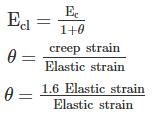θ = 1.6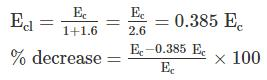% decrease = 61.5%

QUESTION: 7

A rectangular beam of width b = 250 mm, effective depth d = 350 mm is reinforced in tension with mild steel of grade Fe250, Ast = 1800 mm2. The grade of concrete used is M30. The ultimate moment of resistance of the beam is ________

Solution: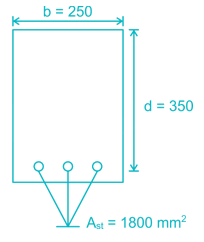Xu,max = 0.53 d= 0.53 × 350 = 185.5 mm

Calculating actual neutral axis of the beam

0.36 fck b Xu = 0.87 fAst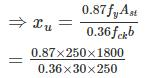= 145 mm < xu,max

∴ Under reinforced section.

∴ Moment of resistance,

Mu = 0.87 fAst (d – 0.42 xu)

= 0.87 × 250 × 1800 (350 – 0.42 × 145)

= 113.182 × 106 N – mm

= 113.182 kN-m

QUESTION: 8

An isolated T-beam used as the walking – way is shown in the figure. The clear span is 5m and fixed at both the ends. Calculated the effective flange width of the T-beam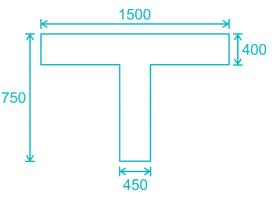Solution:

effective length, lo = 0.7 × 5000 = 3500 mm

for isolated ‘T’ beam, effective flange width,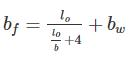where, b = 1500 mm

bw = width of the web = 450 mm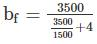+ 450 = 1002.63mm

QUESTION: 9

A doubly reinforced concrete beam with an effective cover of 50 mm to center of both tension and compression reinforcement with an effective depth of 550 mm. If the maximum permissible stress in the outermost fibres in both steel and concrete reaches at the same time, what will be the maximum strain in concrete at the level of compression reinforcement satisfying codal provisions of IS 456:2000 according to limit state method.

Take Fe 500 grade of steel for both tension and compression reinforcement and M 20 grade of concrete.

Solution:

As the maximum permissible stress in the outermost fibers in both steel and concrete reaches at the same time, therefore it is the case of balanced section: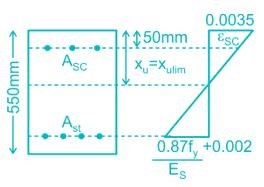So,

Xu = Xulim

For Fe 500

Xulim = 0.46 d

d = effective depth of the beam = 550 mm

Xulim = 0.46 × 550 = 253 mm

d' = effective cover to compression reinforcement = 50 mm

Maximum strain in concrete at outermost compression reinforcement as per IS 456:2000 = 0.0035

Now from similar triangle,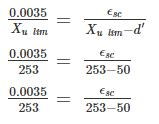ϵsc = 0.00281

QUESTION: 10

A simply supported beam of 300 mm wide and 500 mm deep is loaded as the shear force at support is 140 KN and throughout the beam, the SF does not exceed 70 KN. One out of six bars of 22 mm dia, Fe 415 grade is bent up at 45˚ near the support to resist the shear. Analyze the beam and choose the correct statement: -

M20 grade concrete is used with τ= 0.48Mpa

Solution:

Shear resisted by concrete = τc bd

= 0.48 × 300 × 500 × 10 - 3

= 72 KN > 70 KN

Now, at the support shear to be resisted by bent up bar,

Vus = Vu - τc bd = 140 - 72 = 68 KN

Shear resisted by bent up bars near supports,

Vusb = 0.87 fy Ast Sinα

Vusb = 0.87 × 415 × π/4 × 222 × sin45

= 97 KN > 68 KN

So the beam is safe in shear throughout the beam with bent up bars.

No need of transverse shear reinforcement.